### 1. Definition

A curvilinear traverse is one which includes at least one arc as a side, Figure G-1.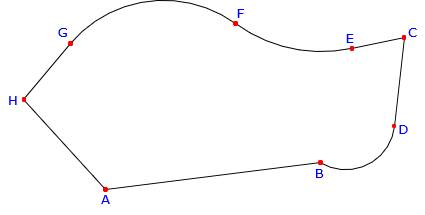Figure G-1 Curvilinear traverse

Many parcel boundaries contain curved lines. Some standard traverse computations need modification in order to account for curves. For example, latitudes and departures are determined for straight lines Understanding a circular arc's geometry and its relationship to adjacent boundary elements is critical to ensure boundaries are correctly defined.

### 2. Arcs

Geometric curved lines are generally sections of circular arcs since those are the most prevalent in horizontal alignments.

#### a. Geometric elements; Nomenclature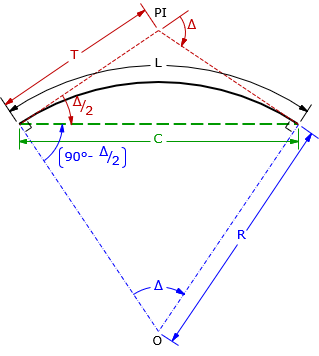PI: Point of Intersection; where the tangent lines intersect Δ: central angle; also the deflection angle between tangents R: radius L: arc length C: chord length T: tangent distance; both tangents are the same length The angle between the tangent and chord is half the central angle: Δ/2 The angle between the chord and radial line at each curve end is (90°-Δ/2) Figure G-2 Arc parts and nomenclature

The primary geometric elements are related through Equations G-1, G-2, and G-3.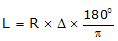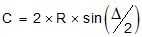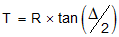Equation G-1 Equation G-2 Equation G-3

When dealing with highway or railroad alignments, an additional geometric element is often specified: degree of curvature, D. There are two different degree of curvature definitions:

• DA, arc definition; the angle subtended by a 100.00' arc, Figure G-3(a)
• DC, chord definition; the angle subtended by a 100.00' chord, Figure G-3(b)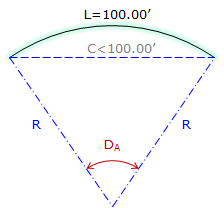(a) Arc definition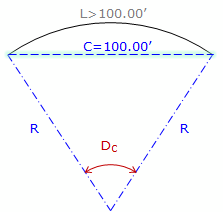(b) Chord definition Figure G-3 Degree of curvature

Arc definition is used in highway and street design while the Chord definition is used in railroad design. Because each definition differs, there is a slightly different radius relationship for each: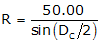Equation G-4 Equation G-5 R in feet, D in deg-min-sec

Degree of curvature is an exclusively English units system attribute as there is no 100.00' equivalent in the metric system. Because of this, we won't use it here in the COGO section.

#### b. Sectors; Segments

Sectors and segments are parts of a circle and are used to compute areas enclosed by curvilinear boundaries.

• A sector is a wedge of a circle bound by the arc and radial lines spaced Δ apart, Figure G-4.
• A  segment is the area between the arc and its chord, Figure G-5.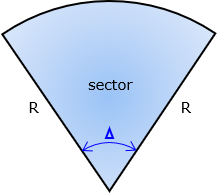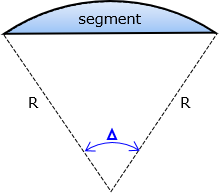Figure G-4 Sector Figure G-5 Segment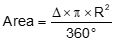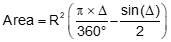Equation G-6 Equation G-7

### 3. Tangency Condition

At its ends, an arc can be joined to a straight line or another arc. At the connection point, the relationship with the adjacent boundary can be tangent or non tangent.

For a tangent condition, Figure G-6:

• Line to arc (and arc to line) - line is perpendicular to the radius at the intersection.
• Arc to Arc - intersection point and radius point of both arcs are collinear; all three point are on a straight line.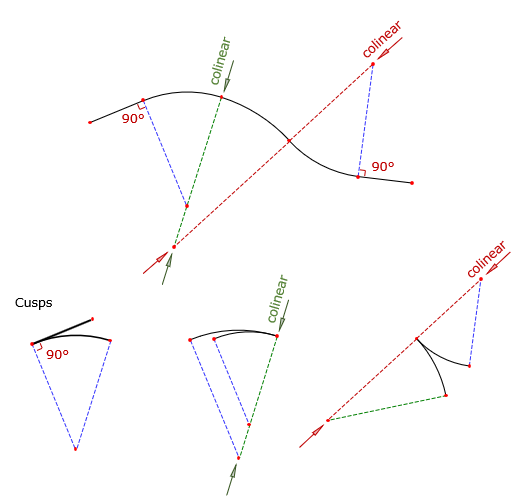Figure G-6 Tangent conditions

Any deviation from those constraints results in a non-tangent transition, Figure G-7.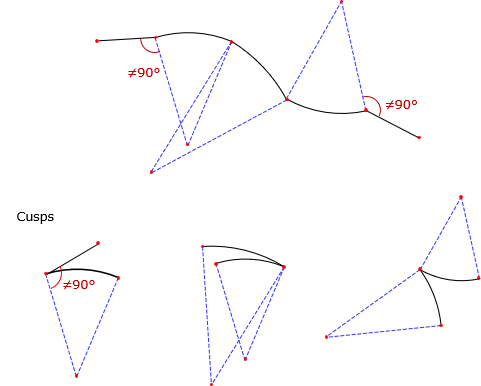Figure G-7 Non-tangent conditions

Tangent transitions (except for cusps) are particularly important in transportation network design. A tangent condition provides a smooth directional transition from one segment to another, important for passenger safety and vehicle dynamics. Non-tangent conditions are generally limited to controlled intersections where the transporting vehicle is brought to a complete stop before changing direction.

A typical subdivision will have a mix of tangent and non-tangent curves. For example, streets are designed using tangent curves while lot lines intersecting streets usually do so in non-tangent conditions, Figure G-8.Figure G-8 Tangent and non-tangent conditions

### 4. Traverse with curves

#### a. Arc incorporation

To incorporate a curve in a traverse requires defining the curve's geometry and its tangency conditions at both ends. To fix an arc's geometry, at least two of its geometric elements (e.g., R, Δ, L, T, C) must be fixed. COGO tools can be used with arc geometry and orientation to compute point positions. This gives us the ability to compute more complex collections of traverses such as Figure G-9 (taken from the Example Plats section of the Wisconsin Platting Manual, Plat Review, Wisconsin Dept of Administration).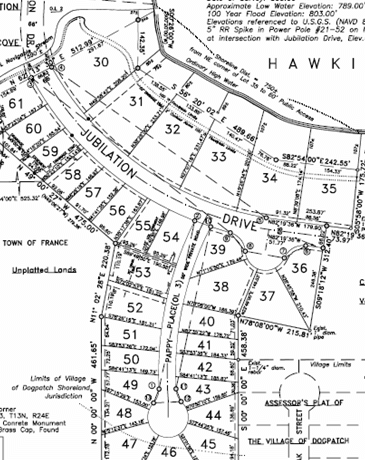Figure G-9 Multiple curvilinear traverse

#### b. Simple curvilinear traverse example

Given the traverse data in Figure G-10.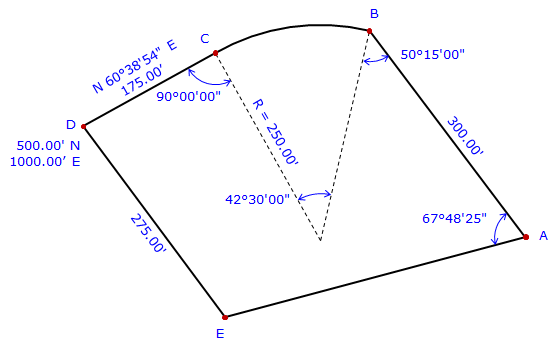Figure G-10 Example

Curve BC is non-tangent at point B, tangent at point C.

Explain how to determine to coordinates of the traverse points.

 We have to start where there are coordinates. In this case, that's at point D. From point D we can go to either point E or point C. There isn't enough information to compute point E's coordinate, but there is to compute point C's. Perform a Forward Computation to point C.From point C we can compute point B's coordinates two ways: through the curve radius point along the curve's chord We'll use the first way: Compute direction from point C to radius point: Az = (60°38’54”+180°)-90°00’00” = 150°38’54” Perform a Forward Computation to radius point, RP.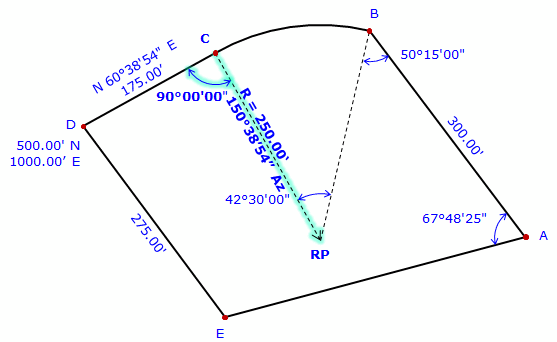At the radius point, compute direction to point B. Az = (150°38'54"+180°00'00") + 42°30'00" = 373°08'54" = 13°08'54" Perform a forward computation to point B.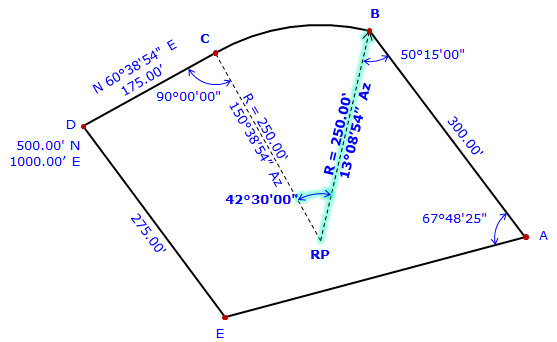At point B, compute direction to point A. Az = (13°08'54"+180°00'00) - 50°15'00" = 142°53'54" Perform a Forward Computation to point A.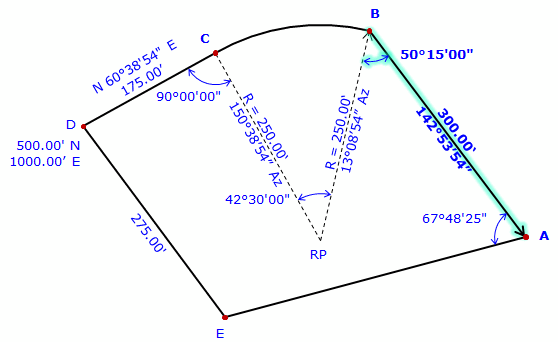There isn't sufficient data to perform a Forward Computation from point A to point E. Point E is also connected to point D but there isn't enough data to perform a Forward Computation from point D. However, point E can be determined by a Direction-distance intersection using its connections to points A and D. Compute direction from point A to E Az = (142°53'54"+180°00'00") - 67°48'25" = 255°05'29" Compute the Direction-distance intersectionHits: 11538